# Mixed g-monotone property and quadruple fixed point theorems in partially ordered G-metric spaces using $\left(\varphi -\psi \right)$ contractions

## Abstract

In this paper, we prove some quadruple coincidence and quadruple common fixed point theorems for $F:{X}^{4}\to X$ and $g:X\to X$ satisfying $\left(\varphi -\psi \right)$ contractions in partially ordered G-metric spaces. We illustrate our results based on an example of the main theorems. Also, we deduce quadruple coincidence points for mappings satisfying the contraction condition of integral type.

MSC:54H25, 47H10, 54E50.

## 1 Introduction and preliminaries

In 1992, Dhage introduced a new class of generalized metric spaces called D-metric spaces (see ). In a subsequent series of papers, Dhage attempted to develop topological structures in such spaces (see ). He claimed that D-metrics provide a generalization of ordinary metric functions and went on to present several fixed point results. In , in collaboration with Sims we demonstrate that most of the claims concerning the fundamental topological structure of D-metric space are incorrect, we also introduce a valid generalized metric space structure, which we call G-metric spaces. Some other papers dealing with G-metric spaces are those in . Recently, there has been growing interest in establishing fixed point theorems in partially ordered complete G-metric spaces with the contractive condition which holds for all points that are related by partial ordering ([18, 19] and ).

In , coupled fixed point results in partially ordered metric spaces were established. After the publication of this work, several coupled fixed point and coincidence point results have appeared in recent literatures (see, for instance, [19, 2237] and ).

Recently, Vasile Berinde and Marin Borcut  extended and generalized the results of  to the case of a contractive operator $F:X×X×X\to X$, where X is a complete ordered metric space. They introduced the concept of a tripled fixed point and the mixed monotone property of a mapping $F:X×X×X\to X$. For more details on tripled fixed point results, we refer the reader to  and .

Very recently, the notion of a fixed point of order $N\ge 3$ was introduced in , and later in  Erdal Karapinar and Nguyen Van Luong introduced the concept of a quadruple fixed point and the mixed monotone property of a mapping $F:X×X×X×X\to X$ and they presented some new fixed point results. Then, a quadruple fixed point is developed and related fixed points are obtained (see ).

In this paper, we prove some quadruple coincidence and quadruple common fixed point theorems for $F:{X}^{4}\to X$ and $g:X\to X$ satisfying $\left(\varphi -\psi \right)$ contractions in partially ordered G-metric spaces. We illustrate our results based on an example of the main theorems. Also, we deduce quadruple coincidence points for mappings satisfying the contraction condition of integral type, we shall recall some mathematical preliminaries.

Definition 1.1 

Let X be a nonempty set, and let $G:X×X×X\to {\mathbf{R}}^{+}$ be a function satisfying the following properties:

(G1) $G\left(x,y,z\right)=0$ if $x=y=z$;

(G2) $0 for all $x,y\in X$ with $x\ne y$;

(G3) $G\left(x,x,y\right)\le G\left(x,y,z\right)$ for all $x,y,z\in X$ with $z\ne y$;

(G4) $G\left(x,y,z\right)=G\left(x,z,y\right)=G\left(y,z,x\right)=\cdots$ (symmetry in all three variables); and

(G5) $G\left(x,y,z\right)\le G\left(x,a,a\right)+G\left(a,y,z\right)$ for all $x,y,z,a\in X$ (rectangle inequality).

Then the function G is called a generalized metric or, more specifically, a G-metric on X, and the pair $\left(X,G\right)$ is called a G-metric space.

Example 1.1 

Let $\left(X,d\right)$ be a usual metric space, and define ${G}_{s}$ and ${G}_{m}$ on $X×X×X$ to ${\mathbf{R}}^{+}$ by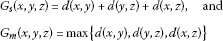for all $x,y,z\in X$. Then $\left(X,{G}_{s}\right)$ and $\left(X,{G}_{m}\right)$ are G-metric spaces.

Definition 1.2 

Let $\left(X,G\right)$ be a G-metric space, and let $\left({x}_{n}\right)$ be a sequence of points of X. A point $x\in X$ is said to be the limit of the sequence $\left({x}_{n}\right)$ if ${lim}_{n,m\to \mathrm{\infty }}G\left(x,{x}_{n},{x}_{m}\right)=0$, and one says that the sequence $\left({x}_{n}\right)$ is G-convergent to x.

Thus, if ${x}_{n}⟶0$ in a G-metric space $\left(X,G\right)$, then for any $ϵ>0$, there exists $N\in \mathbf{N}$ such that $G\left(x,{x}_{n},{x}_{m}\right)<ϵ$ for all $n,m\ge N$ (we mean by N the natural numbers).

Proposition 1.1 

Let $\left(X,G\right)$ be a G-metric space. Then the following are equivalent.

1. (1)

$\left({x}_{n}\right)$ is G-convergent to x.

2. (3)

$G\left({x}_{n},{x}_{n},x\right)\to 0$, as $n\to \mathrm{\infty }$.

3. (4)

$G\left({x}_{n},x,x\right)\to 0$, as $n\to \mathrm{\infty }$.

4. (5)

$G\left({x}_{m},{x}_{n},x\right)\to 0$, as $m,n\to \mathrm{\infty }$.

Definition 1.3 

Let $\left(X,G\right)$ be a G-metric space. A sequence $\left({x}_{n}\right)$ is called G-Cauchy if, given $ϵ>0$, there is $N\in \mathbf{N}$ such that $G\left({x}_{n},{x}_{m},{x}_{l}\right)<ϵ$ for all $n,m,l\ge N$. That is, $G\left({x}_{n},{x}_{m},{x}_{l}\right)⟶0$ as $n,m,l⟶\mathrm{\infty }$.

Proposition 1.2 

In a G-metric space, $\left(X,G\right)$, the following are equivalent.

1. (1)

The sequence $\left({x}_{n}\right)$ is G-Cauchy.

2. (2)

For every $ϵ>0$, there exists $N\in \mathbf{N}$ such that $G\left({x}_{n},{x}_{m},{x}_{m}\right)<ϵ$ for all $n,m\ge N$.

Proposition 1.3 

Let $\left(X,G\right)$ and $\left({X}^{\prime },{G}^{\prime }\right)$ be two G-metric spaces. Then a function $f:X⟶{X}^{\prime }$ is G-continuous at a point $x\in X$ if and only if it is G-sequentially continuous at x; that is, whenever $\left({x}_{n}\right)$ is G-convergent to x, we have $\left(f\left({x}_{n}\right)\right)$ is G-convergent to $f\left(x\right)$.

Definition 1.4 

A G-metric space $\left(X,G\right)$ is called a symmetric G-metric space if $G\left(x,y,y\right)=G\left(y,x,x\right)$ for all $x,y\in X$.

It is clear that any G-metric space where G derives from an underlying metric via ${G}_{s}$ or ${G}_{m}$ in Example 1.1 is symmetric.

Proposition 1.4 

Let $\left(X,G\right)$ be a G-metric space, then the function $G\left(x,y,z\right)$ is jointly continuous in all three of its variables.

Proposition 1.5 

Every G-metric space $\left(X,G\right)$ induces a metric space $\left(X,{d}_{G}\right)$ defined by

${d}_{G}\left(x,y\right)=G\left(x,y,y\right)+G\left(y,x,x\right),\phantom{\rule{1em}{0ex}}\mathrm{\forall }x,y\in X.$

Note that if $\left(X,G\right)$ is symmetric, then

${d}_{G}\left(x,y\right)=2G\left(x,y,y\right),\phantom{\rule{1em}{0ex}}\mathrm{\forall }x,y\in X.$
(1.1)

However, if $\left(X,G\right)$ is not symmetric, then it holds by the G-metric properties that

$\frac{3}{2}G\left(x,y,y\right)\le {d}_{G}\left(x,y\right)\le 3G\left(x,y,y\right),\phantom{\rule{1em}{0ex}}\mathrm{\forall }x,y\in X.$
(1.2)

Definition 1.5 

A G-metric space $\left(X,G\right)$ is said to be G-complete (or complete G-metric) if every G-Cauchy sequence in $\left(X,G\right)$ is G-convergent in $\left(X,G\right)$.

Definition 1.6 Let $\left(X,G\right)$ be a G-metric space. A mapping $F:X×X×X×X\to X$ is said to be continuous if for any G-convergent sequences $\left\{{x}_{n}\right\}$, $\left\{{y}_{n}\right\}$, $\left\{{z}_{n}\right\}$, and $\left\{{w}_{n}\right\}$ converging to x, y, z, and w respectively, $\left\{F\left({x}_{n},{y}_{n},{z}_{n},{w}_{n}\right)\right\}$ is G-convergent to $F\left(x,y,z,w\right)$.

Proposition 1.6 

A G-metric space $\left(X,G\right)$ is G-complete if and only if $\left(X,{d}_{G}\right)$ is a complete metric space.

Following Erdal , we introduce the following definitions.

Definition 1.7  Let X be a nonempty set and $F:X×X×X×X\to X$ be a given mapping. An element $\left(x,y,z,w\right)\in X×X×X×X$ is called a quadruple fixed point of F ifDefinition 1.8  Let $\left(X,\le \right)$ be a partially ordered set and $F:X×X×X×X\to X$ be a mapping. We say that F has the mixed monotone property if $F\left(x,y,z,w\right)$ is monotone non-decreasing in x and z and is monotone non-increasing in y and w; that is, for any $x,y,z,w\in X$,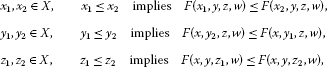and

${w}_{1},{w}_{2}\in X,\phantom{\rule{2em}{0ex}}{w}_{1}\le {w}_{2}\phantom{\rule{1em}{0ex}}\text{implies}\phantom{\rule{1em}{0ex}}F\left(x,y,z,{w}_{2}\right)\le F\left(x,y,z,{w}_{1}\right).$

Definition 1.9  Let X be a non-empty set. Then we say that the mappings $F:{X}^{4}\to X$ and $g:X\to X$ are commutative if for all $x,y,z,w\in X$,

$g\left(F\left(x,y,z,w\right)\right)=F\left(gx,gy,gz,gw\right).$

Definition 1.10  Let $\left(X,\le \right)$ be a partially ordered set. Let $F:{X}^{4}\to X$ and $g:X\to X$. The mapping F is said to have the mixed g-monotone property if for any $x,y,z,w\in X$,Definition 1.11  Let $F:{X}^{4}\to X$ and $g:X\to X$. An element $\left(x,y,z,w\right)$ is called a quadruple coincidence point of F and g if

$F\left(x,y,z,w\right)=gx,\phantom{\rule{2em}{0ex}}F\left(y,z,w,x\right)=gy,\phantom{\rule{2em}{0ex}}F\left(z,w,x,y\right)=gz,\phantom{\rule{1em}{0ex}}\text{and}\phantom{\rule{1em}{0ex}}F\left(w,x,y,z\right)=gw.$

$\left(gx,gy,gz,gw\right)$ is said to be a quadruple point of coincidence of F and g.

Definition 1.12  Let $F:{X}^{4}\to X$ and $g:X\to X$. An element $\left(x,y,z,w\right)$ is called a quadruple common fixed point of F and g ifLet Φ be the set of all functions $\varphi :\left[0,+\mathrm{\infty }\right)\to \left[0,+\mathrm{\infty }\right)$ which satisfy

1. (1)

ϕ is continuous and non-decreasing;

2. (2)

$\varphi \left(t\right)=0$ iff $t=0$;

3. (3)

$\varphi \left(t+s\right)\le \varphi \left(t\right)+\varphi \left(s\right)$, $\mathrm{\forall }t,s\in \left[0,\mathrm{\infty }\right)$.

And let Ψ denote all functions $\psi :\left[0,+\mathrm{\infty }\right)\to \left[0,+\mathrm{\infty }\right)$ which satisfy

1. (1)

${lim}_{t\to r}\psi \left(t\right)>0$ for all $r>0$, and

2. (2)

${lim}_{t\to +0}\psi \left(t\right)=0$.

For example , the functions ${\varphi }_{1}\left(t\right)=kt$, $k>0$, ${\varphi }_{2}\left(t\right)=\frac{t}{1+t}$ are in Φ and ${\psi }_{1}\left(t\right)=kt$, $k>0$, ${\psi }_{2}\left(t\right)=\frac{ln\left(2k+1\right)}{2}$ are in Ψ.

Remark 1 $\mathrm{\Phi }\subseteq \mathrm{\Psi }$.

Remark 2 For all $t\in \left[0,+\mathrm{\infty }\right)$, we have $\frac{1}{2}\varphi \left(t\right)\le \varphi \left(\frac{t}{2}\right)$.

## 2 Main results

Theorem 2.1 Let $\left(X,\le \right)$ be a partially ordered set and $\left(X,G\right)$ be a G-metric space. Let $F:X×X×X×X\to X$ and $g:X\to X$ be such that F has the mixed g-monotone property. Assume that there exist $\varphi \in \mathrm{\Phi }$ and $\psi \in \mathrm{\Psi }$ such that(2.1)

for all $x,y,z,w,u,v,s,t,a,b,c,d\in X$ with $gx\ge gu\ge ga$, $gy\le gv\le gb$, $gz\ge gs\ge gc$, and $gw\le gt\le gd$. Suppose $F\left({X}^{4}\right)\subseteq g\left(X\right)$, g is continuous and commutes with F. If there exist ${x}_{0},{y}_{0},{z}_{0},{w}_{0}\in X$ such thatsuppose either

1. (a)

$\left(X,G\right)$ is a complete G-metric space and F is continuous or

2. (b)

$\left(g\left(X\right),G\right)$ is complete and $\left(X,G,\le \right)$ has the following property: then there exist $x,y,z,w\in X$ such that

$F\left(x,y,z,w\right)=gx,\phantom{\rule{2em}{0ex}}F\left(y,z,w,x\right)=gy,\phantom{\rule{2em}{0ex}}F\left(z,w,x,y\right)=gz,\phantom{\rule{1em}{0ex}}\mathit{\text{and}}\phantom{\rule{1em}{0ex}}F\left(w,x,y,z\right)=gw,$

that is, F and g have a quadruple coincidence point.

1. (i)

if a non-decreasing sequence ${x}_{n}\to a$, then ${x}_{n}\le x$ for all n,

2. (ii)

if a non-increasing sequence ${y}_{n}\to y$, then $y\le {y}_{n}$ for all n,

Proof Let ${x}_{0},{y}_{0},{z}_{0},{w}_{0}\in X$ be such thatSince $F\left({X}^{4}\right)\subset g\left(X\right)$, then we can choose ${x}_{1},{y}_{1},{z}_{1},{w}_{1}\in X$ such that

$\begin{array}{r}g{x}_{1}=F\left({x}_{0},{y}_{0},{z}_{0},{w}_{0}\right),\phantom{\rule{2em}{0ex}}g{y}_{1}=F\left({y}_{0},{z}_{0},{w}_{0},{x}_{0}\right),\\ g{z}_{1}=F\left({z}_{0},{w}_{0},{x}_{0},{y}_{0}\right),\phantom{\rule{1em}{0ex}}\text{and}\phantom{\rule{1em}{0ex}}g{w}_{1}=F\left({w}_{0},{x}_{0},{y}_{0},{z}_{0}\right).\end{array}$
(2.2)

Taking into account $F\left({X}^{4}\right)\subset g\left(X\right)$, by continuing this process, we can construct sequences $\left\{{x}_{n}\right\},\left\{{y}_{n}\right\}$, $\left\{{z}_{n}\right\}$, and $\left\{{w}_{n}\right\}$ in X such that

$\begin{array}{r}g{x}_{n+1}=F\left({x}_{n},{y}_{n},{z}_{n},{w}_{n}\right),\phantom{\rule{2em}{0ex}}g{y}_{n+1}=F\left({y}_{n},{z}_{n},{w}_{n},{x}_{n}\right),\\ g{z}_{n+1}=F\left({z}_{n},{w}_{n},{x}_{n},{y}_{n}\right),\phantom{\rule{1em}{0ex}}\text{and}\phantom{\rule{1em}{0ex}}g{w}_{n+1}=F\left({w}_{n},{x}_{n},{y}_{n},{z}_{n}\right).\end{array}$
(2.3)

We shall show that

(2.4)

For this purpose, we use the mathematical induction. Since $g{x}_{0}\le F\left({x}_{0},{y}_{0},{z}_{0},{w}_{0}\right)$, $g{y}_{0}\ge F\left({y}_{0},{z}_{0},{w}_{0},{x}_{0}\right)$, $g{z}_{0}\le F\left({z}_{0},{w}_{0},{x}_{0},{y}_{0}\right)$, and $g{w}_{0}\ge F\left({w}_{0},{x}_{0},{y}_{0},{z}_{0}\right)$, then by (2.2), we get

$g{x}_{0}\le g{x}_{1},\phantom{\rule{2em}{0ex}}g{y}_{1}\le g{y}_{0},\phantom{\rule{2em}{0ex}}g{z}_{0}\le g{z}_{1},\phantom{\rule{1em}{0ex}}\text{and}\phantom{\rule{1em}{0ex}}g{w}_{1}\le g{w}_{0},$

that is, (2.4) holds for $n=0$.

We presume that (2.4) holds for some $n>0$. As F has the mixed g-monotone property and $g{x}_{n}\le g{x}_{n+1}$, $g{y}_{n+1}\le g{y}_{n}$, $g{z}_{n}\le g{z}_{n+1}$, and $g{w}_{n+1}\le g{w}_{n}$, we obtainand

$\begin{array}{rcl}g{w}_{n+2}& =& F\left({w}_{n+1},{x}_{n+1},{y}_{n+1},{z}_{n+1}\right)\le F\left({w}_{n+1},{x}_{n},{y}_{n+1},{z}_{n+1}\right)\\ \le & F\left({w}_{n},{x}_{n},{y}_{n+1},{z}_{n+1}\right)\le F\left({w}_{n},{x}_{n},{y}_{n},{z}_{n+1}\right)\\ \le & F\left({w}_{n},{x}_{n},{y}_{n},{z}_{n}\right)=g{w}_{n+1}.\end{array}$

Thus, (2.4) holds for any $n\in \mathbb{N}$. Assume for some $n\in \mathbb{N}$,

$g{x}_{n}=g{x}_{n+1},\phantom{\rule{2em}{0ex}}g{y}_{n}=g{y}_{n+1},\phantom{\rule{2em}{0ex}}g{z}_{n}=g{z}_{n+1},\phantom{\rule{1em}{0ex}}\text{and}\phantom{\rule{1em}{0ex}}g{w}_{n}=g{w}_{n+1}$

then by (2.3), we have $g{x}_{n}=F\left({x}_{n},{y}_{n},{z}_{n},{w}_{n}\right)$, $g{y}_{n}=F\left({y}_{n},{z}_{n},{w}_{n},{x}_{n}\right)$, $g{z}_{n}=F\left({z}_{n},{w}_{n},{x}_{n},{y}_{n}\right)$, and $g{w}_{n}=F\left({w}_{n},{x}_{n},{y}_{n},{z}_{n}\right)⇒\left({x}_{n},{y}_{n},{z}_{n},{w}_{n}\right)$ is a quadruple coincidence point of F and g. From now on, assume for any $n\in \mathbb{N}$ that at least

$g{x}_{n}\ne g{x}_{n+1}\phantom{\rule{1em}{0ex}}\text{or}\phantom{\rule{1em}{0ex}}g{y}_{n}\ne g{y}_{n+1}\phantom{\rule{1em}{0ex}}\text{or}\phantom{\rule{1em}{0ex}}g{z}_{n}\ne g{z}_{n+1}\phantom{\rule{1em}{0ex}}\text{or}\phantom{\rule{1em}{0ex}}g{w}_{n}\ne g{w}_{n+1}.$
(2.5)

Since $g{x}_{n}\le g{x}_{n+1}$, $g{y}_{n+1}\le g{y}_{n}$, $g{z}_{n}\le g{z}_{n+1}$, and $g{w}_{n+1}\le g{w}_{n}$, then from (2.1) and (2.3), we have(2.6)(2.7)(2.8)

and(2.9)

From (2.6), (2.7), (2.8), and (2.9) it follows that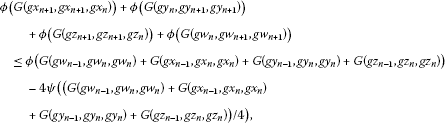(2.10)

but from the property (3) of ϕ, we get $\varphi \left(A+B+C+D\right)\le \varphi \left(A\right)+\varphi \left(B\right)+\varphi \left(C\right)+\varphi \left(D\right)$; therefore,(2.11)

which implies that(2.12)

Using the fact that ϕ is non-decreasing, we get(2.13)

Let ${\delta }_{n}=G\left(g{x}_{n+1},g{x}_{n+1},g{x}_{n}\right)+G\left(g{y}_{n},g{y}_{n+1},g{y}_{n+1}\right)+G\left(g{z}_{n+1},g{z}_{n+1},g{z}_{n}\right)+G\left(g{w}_{n},g{w}_{n+1},g{w}_{n+1}\right)$.

Then the sequence $\left({\delta }_{n}\right)$ is decreasing; therefore, there is some $\delta \ge 0$ such that

$\begin{array}{rcl}\underset{n\to \mathrm{\infty }}{lim}{\delta }_{n}& =& lim\left(G\left(g{x}_{n+1},g{x}_{n+1},g{x}_{n}\right)+G\left(g{y}_{n},g{y}_{n+1},g{y}_{n+1}\right)\\ +G\left(g{z}_{n+1},g{z}_{n+1},g{z}_{n}\right)+G\left(g{w}_{n},g{w}_{n+1},g{w}_{n+1}\right)\right)=\delta .\end{array}$
(2.14)

We will show that $\delta =0$. Suppose to the contrary that $\delta >0$, taking the limit as $n\to \mathrm{\infty }$ of both sides of (1.3) and using the fact that ϕ is continuous and ${lim}_{t\to r}\psi \left(t\right)>0$ for $r>0$, we have

$\varphi \left(\delta \right)=\underset{n\to \mathrm{\infty }}{lim}\varphi \left({\delta }_{n}\right)\le \underset{n\to \mathrm{\infty }}{lim}\left[\varphi \left({\delta }_{n-1}\right)-4\psi \left(\frac{{\delta }_{n-1}}{4}\right)\right]=\varphi \left(\delta \right)-4\underset{n\to \mathrm{\infty }}{lim}\psi \left(\frac{{\delta }_{n-1}}{4}\right)<\varphi \left(\delta \right),$

a contradiction. Thus, $\delta =0$, that is,

$\begin{array}{rcl}\underset{n\to \mathrm{\infty }}{lim}{\delta }_{n}& =& lim\left(G\left(g{x}_{n+1},g{x}_{n+1},g{x}_{n}\right)+G\left(g{y}_{n},g{y}_{n+1},g{y}_{n+1}\right)\\ +G\left(g{z}_{n+1},g{z}_{n+1},g{z}_{n}\right)+G\left(g{w}_{n},g{w}_{n+1},g{w}_{n+1}\right)\right)=0.\end{array}$
(2.15)

We will show that the sequences $\left(g{x}_{n}\right)$, $\left(g{y}_{n}\right)$, $\left(g{z}_{n}\right)$, and $\left(g{w}_{n}\right)$ are G-Cauchy sequences in $\left(X,G\right)$.

Suppose to the contrary that at least one of $\left(g{x}_{n}\right)$, $\left(g{y}_{n}\right)$, $\left(g{z}_{n}\right)$, and $\left(g{w}_{n}\right)$ is not a G-Cauchy sequence, so there exists $ϵ>0$ for which we can find sequences $\left(g{x}_{n\left(k\right)}\right);\left(g{x}_{m\left(k\right)}\right)$ of $\left(g{x}_{n}\right)$, $\left(g{y}_{n\left(k\right)}\right);\left(g{y}_{m\left(k\right)}\right)$ of $\left(g{y}_{n}\right)$, $\left(g{z}_{n\left(k\right)}\right);\left(g{z}_{m\left(k\right)}\right)$ of $\left(g{z}_{n}\right)$, and $\left(g{w}_{n\left(k\right)}\right);\left(g{w}_{m\left(k\right)}\right)$ of $\left(g{w}_{n}\right)$ with $n\left(k\right)>m\left(k\right)\ge k$ such that(2.16)

Further, corresponding to $m\left(k\right)$, we may choose $n\left(k\right)$ such that it is the smallest integer satisfying (2.16) and $n\left(k\right)>m\left(k\right)\ge k$.

Thus,(2.17)

Using the rectangle inequality and having in mind (2.16) and (2.17), we get

$\begin{array}{rcl}ϵ& \le & {t}_{k}\\ =& G\left(g{x}_{n\left(k\right)},g{x}_{n\left(k\right)},g{x}_{m\left(k\right)}\right)+G\left(g{y}_{n\left(k\right)},g{y}_{n\left(k},g{y}_{m\left(k\right)}\right)+G\left(g{z}_{n\left(k\right)},g{z}_{n\left(k\right)},g{z}_{m\left(k\right)}\right)\\ +G\left(g{w}_{n\left(k\right)},g{w}_{n\left(k\right)},g{w}_{m\left(k\right)}\right)\\ \le & G\left(g{x}_{n\left(k\right)},g{x}_{n\left(k\right)},g{x}_{n\left(k\right)-1}\right)+G\left(g{x}_{n\left(k\right)-1},g{x}_{n\left(k\right)-1},g{x}_{m\left(k\right)}\right)+G\left(g{y}_{n\left(k\right)},g{y}_{n\left(k\right)},g{y}_{n\left(k\right)-1}\right)\\ +G\left(g{y}_{n\left(k\right)-1},g{y}_{n\left(k\right)-1},g{y}_{m\left(k\right)}\right)+G\left(g{z}_{n\left(k\right)},g{z}_{n\left(k\right)},g{z}_{n\left(k\right)-1}\right)+G\left(g{z}_{n\left(k\right)-1},g{z}_{n\left(k\right)-1},g{z}_{m\left(k\right)}\right)\\ +G\left(g{w}_{n\left(k\right)},g{w}_{n\left(k\right)},g{w}_{n\left(k\right)-1}\right)+G\left(g{w}_{n\left(k\right)-1},g{w}_{n\left(k\right)-1},g{w}_{m\left(k\right)}\right)\\ <& G\left(g{x}_{n\left(k\right)},g{x}_{n\left(k\right)},g{x}_{n\left(k\right)-1}\right)+G\left(g{y}_{n\left(k\right)},g{y}_{n\left(k\right)},g{y}_{n\left(k\right)-1}\right)+G\left(g{z}_{n\left(k\right)},g{z}_{n\left(k\right)},g{z}_{n\left(k\right)-1}\right)\\ +G\left(g{w}_{n\left(k\right)},g{w}_{n\left(k\right)},g{w}_{n\left(k\right)-1}\right)+ϵ=ϵ+{\delta }_{n\left(k\right)-1}.\end{array}$
(2.18)

Letting $k\to \mathrm{\infty }$ in (2.18) and using $\left(\delta =0\right)$, we get

$\begin{array}{rcl}lim{t}_{k}& =& lim\left(G\left(g{x}_{n\left(k\right)},g{x}_{n\left(k\right)},g{x}_{m\left(k\right)}\right)+G\left(g{y}_{n\left(k\right)},g{y}_{n\left(k\right)},g{y}_{m\left(k\right)}\right)\\ +G\left(g{z}_{n\left(k\right)},g{z}_{n\left(k\right)},g{z}_{m\left(k\right)}\right)+G\left(g{w}_{n\left(k\right)},g{w}_{n\left(k\right)},g{w}_{m\left(k\right)}\right)\right)=ϵ.\end{array}$
(2.19)

Again by the rectangle inequality and using the fact that $G\left(x,y,y\right)\le 2G\left(y,x,x\right)$, we have

$\begin{array}{rcl}ϵ& \le & {t}_{k}\\ =& G\left(g{x}_{n\left(k\right)},g{x}_{n\left(k\right)},g{x}_{m\left(k\right)}\right)+G\left(g{y}_{n\left(k\right)},g{y}_{n\left(k\right)},g{y}_{m\left(k\right)}\right)+G\left(g{z}_{n\left(k\right)},g{z}_{n\left(k\right)},g{z}_{m\left(k\right)}\right)\\ +G\left(g{w}_{n\left(k\right)},g{w}_{n\left(k\right)},{w}_{m\left(k\right)}\right)\\ \le & G\left(g{x}_{n\left(k\right)},g{x}_{n\left(k\right)},g{x}_{n\left(k\right)+1}\right)+G\left(g{x}_{n\left(k\right)+1},g{x}_{n\left(k\right)+1},g{x}_{m\left(k\right)+1}\right)+G\left(g{x}_{m\left(k\right)+1},g{x}_{m\left(k\right)+1},g{x}_{m\left(k\right)}\right)\\ +G\left(g{y}_{n\left(k\right)},g{y}_{n\left(k\right)},g{y}_{n\left(k\right)+1}\right)+G\left(g{y}_{n\left(k\right)+1},g{y}_{n\left(k\right)+1},g{y}_{m\left(k\right)+1}\right)\\ +G\left(g{y}_{m\left(k\right)+1},g{y}_{m\left(k\right)+1},g{y}_{m\left(k\right)}\right)+G\left(g{z}_{n\left(k\right)},g{z}_{n\left(k\right)},g{z}_{n\left(k\right)+1}\right)\\ +G\left(g{z}_{n\left(k\right)+1},g{z}_{n\left(k\right)+1},g{z}_{m\left(k\right)+1}\right)+G\left(g{z}_{m\left(k\right)+1},g{z}_{m\left(k\right)+1},g{z}_{m\left(k\right)}\right)\\ +G\left(g{w}_{n\left(k\right)},g{w}_{n\left(k\right)},g{w}_{n\left(k\right)+1}\right)+G\left(g{w}_{n\left(k\right)+1},g{w}_{n\left(k\right)+1},g{w}_{m\left(k\right)+1}\right)\\ +G\left(g{w}_{m\left(k\right)+1},g{w}_{m\left(k\right)+1},g{w}_{m\left(k\right)}\right)\\ \le & 2{\delta }_{n\left(k\right)}+{\delta }_{m\left(k\right)}+G\left(g{x}_{n\left(k\right)+1},g{x}_{n\left(k\right)+1},g{x}_{m\left(k\right)+1}\right)\\ +G\left(g{y}_{n\left(k\right)+1},g{y}_{n\left(k\right)+1},g{y}_{m\left(k\right)+1}\right)+G\left(g{z}_{n\left(k\right)+1},g{z}_{n\left(k\right)+1},g{z}_{m\left(k\right)+1}\right)\\ +G\left(g{w}_{n\left(k\right)+1},g{w}_{n\left(k\right)+1},g{w}_{m\left(k\right)+1}\right).\end{array}$
(2.20)

Using the property of ϕ, we have

$\begin{array}{rcl}\varphi \left({t}_{k}\right)& =& \varphi \left[G\left(g{x}_{n\left(k\right)},g{x}_{n\left(k\right)},g{x}_{m\left(k\right)}\right)+G\left(g{y}_{n\left(k\right)},g{y}_{n\left(k\right)},g{y}_{m\left(k\right)}\right)+G\left(g{z}_{n\left(k\right)},g{z}_{n\left(k\right)},g{z}_{m\left(k\right)}\right)\\ +G\left(g{w}_{n\left(k\right)},g{w}_{n\left(k\right)},{w}_{m\left(k\right)}\right)\right]\\ \le & \varphi \left(2{\delta }_{n\left(k\right)}+{\delta }_{m\left(k\right)}\right)+\varphi \left(G\left(g{x}_{n\left(k\right)+1},g{x}_{n\left(k\right)+1},g{x}_{m\left(k\right)+1}\right)\right)\\ +\varphi \left(G\left(g{y}_{n\left(k\right)+1},g{y}_{n\left(k\right)+1},g{y}_{m\left(k\right)+1}\right)\right)+\varphi \left(G\left(g{z}_{n\left(k\right)+1},g{z}_{n\left(k\right)+1},g{z}_{m\left(k\right)+1}\right)\right)\\ +\varphi \left(G\left(g{w}_{n\left(k\right)+1},g{w}_{n\left(k\right)+1},g{w}_{m\left(k\right)+1}\right)\right).\end{array}$
(2.21)

Since $n\left(k\right)>m\left(k\right)$, then $g{x}_{n\left(k\right)}\ge g{x}_{m\left(k\right)}$, $g{y}_{n\left(k\right)}\le g{x}_{m\left(k\right)}$, $g{z}_{n\left(k\right)}\ge g{z}_{m\left(k\right)}$, and $g{w}_{n\left(k\right)}\le g{w}_{m\left(k\right)}$.

Then from (2.1), we have(2.22)

and similarly,(2.23)(2.24)

and(2.25)

Combining (2.22), (2.23), (2.24), and (2.25) in (2.21), we get

$\varphi \left({t}_{k}\right)\le \varphi \left(2{\delta }_{n\left(k\right)}+{\delta }_{m\left(k\right)}\right)+\varphi \left({t}_{k}\right)-4\psi \left(\frac{{t}_{k}}{4}\right).$

Letting, $k\to \mathrm{\infty }$ and using (2.15) and (2.19), we get

$\varphi \left(ϵ\right)\le \varphi \left(0\right)+\varphi \left(0\right)+\varphi \left(ϵ\right)-4\underset{k\to \mathrm{\infty }}{lim}\psi \left(\frac{{t}_{k}}{4}\right)$
(2.26)
$=\varphi \left(ϵ\right)-4\underset{{t}_{k}\to ϵ}{lim}\psi \left(\frac{{t}_{k}}{4}\right)<\varphi \left(ϵ\right),$
(2.27)

a contradiction. This implies that $\left(g{x}_{n}\right)$, $\left(g{y}_{n}\right)$, $\left(g{z}_{n}\right)$, and $\left(g{w}_{n}\right)$ are G-Cauchy sequences in $\left(X,G\right)$.

Now suppose that the assumption (a) holds.

Since X is a G-complete metric space, there exist $x,y,z,w\in X$ such that

$\begin{array}{r}\underset{n\to \mathrm{\infty }}{lim}g\left({x}_{n}\right)=x,\phantom{\rule{2em}{0ex}}\underset{n\to \mathrm{\infty }}{lim}g\left({y}_{n}\right)=y,\\ \underset{n\to \mathrm{\infty }}{lim}g\left({z}_{n}\right)=z,\phantom{\rule{2em}{0ex}}\underset{n\to \mathrm{\infty }}{lim}g\left({w}_{n}\right)=w.\end{array}$
(2.28)

From (2.28) and the continuity of g, we haveFrom the commutativity of F and g, we have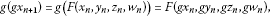(2.29)(2.30)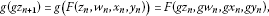(2.31)

and

$g\left(g{w}_{n+1}\right)=g\left(F\left({w}_{n},{x}_{n},{y}_{n},{z}_{n}\right)\right)=F\left(g{w}_{n},g{x}_{n},g{y}_{n},g{z}_{n}\right).$
(2.32)

We shall show that $gx=F\left(x,y,z,w\right)$, $gy=F\left(y,z,w,x\right)$, $gz=F\left(z,w,x,y\right)$, and $gw=F\left(w,x,y,z\right)$.

By letting $n\to \mathrm{\infty }$ in (2.29) → (2.32) and using the continuity of F, we obtain

$\begin{array}{rcl}gx& =& \underset{n\to \mathrm{\infty }}{lim}g\left(g{x}_{n+1}\right)=\underset{n\to \mathrm{\infty }}{lim}F\left(g{x}_{n},g{y}_{n},g{z}_{n},g{w}_{n}\right)\\ =& F\left(\underset{n\to \mathrm{\infty }}{lim}g{x}_{n},\underset{n\to \mathrm{\infty }}{lim}g{y}_{n},\underset{n\to \mathrm{\infty }}{lim}g{z}_{n},\underset{n\to \mathrm{\infty }}{lim}g{w}_{n}\right)=F\left(x,y,z,w\right).\end{array}$

Similarly, $gy=F\left(y,z,w,x\right)$, $gz=F\left(z,w,x,y\right)$, and $gw=F\left(w,x,y,z\right)$.

Hence, $\left(x,y,z,w\right)$ is a coincidence point of F and g.

Now suppose that the assumption (b) holds.

Since $\left\{g{x}_{n}\right\}$, $\left\{g{y}_{n}\right\}$, $\left\{g{z}_{n}\right\}$, and $\left\{g{w}_{n}\right\}$ are G-Cauchy sequences in the complete G-metric space $\left(g\left(X\right),G\right)$, then there exist $x,y,z,w\in X$ such that

$g{x}_{n}\to gx,\phantom{\rule{2em}{0ex}}g{y}_{n}\to gy,\phantom{\rule{2em}{0ex}}g{z}_{n}\to gz,\phantom{\rule{1em}{0ex}}\text{and}\phantom{\rule{1em}{0ex}}g{w}_{n}\to gw.$
(2.33)

Since $\left\{g{x}_{n}\right\}$, $\left\{g{z}_{n}\right\}$ are non-decreasing and $\left\{g{y}_{n}\right\}$, $\left\{g{w}_{n}\right\}$ are non-increasing and since $\left(X,G,\le \right)$ satisfies conditions (i) and (ii), we have

If $g{x}_{n}=gx$, $g{y}_{n}=gy$, $g{z}_{n}=gz$, and $g{w}_{n}=gw$ for some $n\ge 0$, then $gx=g{x}_{n}\le g{x}_{n+1}\le gx=g{x}_{n}$, $gy\le g{y}_{n+1}\le g{y}_{n}=gy$, $gz=g{z}_{n}\le g{z}_{n+1}\le gz=g{z}_{n}$, and $gw\le g{w}_{n+1}\le g{w}_{n}=gw$, which implies that

$g{x}_{n}=g{x}_{n+1}=F\left({x}_{n},{y}_{n},{z}_{n},{w}_{n}\right),\phantom{\rule{2em}{0ex}}g{y}_{n}=g{y}_{n+1}=F\left({y}_{n},{z}_{n},{w}_{n},{x}_{n}\right),$

and

$g{z}_{n}=g{z}_{n+1}=F\left({z}_{n},{w}_{n},{x}_{n},{y}_{n}\right),\phantom{\rule{2em}{0ex}}g{w}_{n}=g{w}_{n+1}=F\left({w}_{n},{w}_{n},{y}_{n},{z}_{n}\right),$

that is, $\left({x}_{n},{y}_{n},{z}_{n},{w}_{n}\right)$ is a quadruple coincidence point of F and g. Then, we suppose that $\left(g{x}_{n},g{y}_{n},g{z}_{n},g{w}_{n}\right)\ne \left(gx,gy,gz,gw\right)$ for all $n\ge 0$. By (2.1), consider now(2.34)

Taking the limit as $n\to \mathrm{\infty }$ in (2.34) and using the property of ϕ, hence we get that $G\left(gx,F\left(x,y,z,w\right),F\left(x,y,z,w\right)\right)=0$. Thus, $gx=F\left(x,y,z,w\right)$. Analogously, one finds

$F\left(y,z,w,x\right)=gy,\phantom{\rule{2em}{0ex}}F\left(z,w,x,y\right)=gz,\phantom{\rule{1em}{0ex}}\text{and}\phantom{\rule{1em}{0ex}}F\left(w,x,y,z\right)=gw.$

Thus, we proved that F and g have a quadruple coincidence point. This completes the proof of Theorem 2.1. □

Corollary 2.1 Let $\left(X,\le \right)$ be a partially ordered set and $\left(X,G\right)$ be a G-metric space. Let $F:X×X×X×X\to X$ and $g:X\to X$ be such that F has the mixed g-monotone property. Assume that there exists $\psi \in \mathrm{\Psi }$ such that(2.35)

for all $x,y,z,w,u,v,s,t,a,b,c,d\in X$ with $gx\ge gu\ge ga$, $gy\le gv\le gb$, $gz\ge gs\ge gc$, and $gw\le gt\le gd$. Suppose $F\left({X}^{4}\right)\subseteq g\left(X\right)$, g is continuous and commutes with F. If there exist ${x}_{0},{y}_{0},{z}_{0},{w}_{0}\in X$ such thatsuppose either

1. (a)

$\left(X,G\right)$ is a complete G-metric space and F is continuous or

2. (b)

$\left(g\left(X\right),G\right)$ is complete and $\left(X,G,\le \right)$ has the following property: then there exist $x,y,z,w\in X$ such that

$F\left(x,y,z,w\right)=gx,\phantom{\rule{2em}{0ex}}F\left(y,z,w,x\right)=gy,\phantom{\rule{2em}{0ex}}F\left(z,w,x,y\right)=gz,\phantom{\rule{1em}{0ex}}\mathit{\text{and}}\phantom{\rule{1em}{0ex}}F\left(w,x,y,z\right)=gw,$

that is, F and g have a quadruple coincidence point.

1. (i)

if a non-decreasing sequence ${x}_{n}\to a$, then ${x}_{n}\le x$ for all n,

2. (ii)

if a non-increasing sequence ${y}_{n}\to y$, then $y\le {y}_{n}$ for all n,

Proof In Theorem 2.1 taking $\varphi \left(t\right)=t$, we get Corollary 2.1. □

Corollary 2.2 Let $\left(X,\le \right)$ be a partially ordered set and $\left(X,G\right)$ be a G-metric space. Let $F:X×X×X×X\to X$ and $g:X\to X$ be such that F has the mixed g-monotone property such that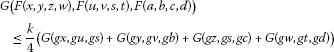for all $x,y,z,w,u,v,s,t,a,b,c,d\in X$ with $gx\ge gu\ge ga$, $gy\le gv\le gb$, $gz\ge gs\ge gc$, and $gw\le gt\le gd$. Suppose $F\left({X}^{4}\right)\subseteq g\left(X\right)$, g is continuous and commutes with F. If there exist ${x}_{0},{y}_{0},{z}_{0},{w}_{0}\in X$ such thatsuppose either

1. (a)

$\left(X,G\right)$ is a complete G-metric space and F is continuous or

2. (b)

$\left(X,G,\le \right)$ has the following property: then there exist $x,y,z,w\in X$ such that

$F\left(x,y,z,w\right)=gx,\phantom{\rule{2em}{0ex}}F\left(y,z,w,x\right)=gy,\phantom{\rule{2em}{0ex}}F\left(z,w,x,y\right)=gz,\phantom{\rule{1em}{0ex}}\mathit{\text{and}}\phantom{\rule{1em}{0ex}}F\left(w,x,y,z\right)=gw,$

that is, F and g have a quadruple coincidence point.

1. (i)

if a non-decreasing sequence ${x}_{n}\to a$, then ${x}_{n}\le x$ for all n,

2. (ii)

if a non-increasing sequence ${y}_{n}\to y$, then $y\le {y}_{n}$ for all n,

Proof In Corollary 2.1 taking $\psi \left(t\right)=\frac{1-k}{4}t$, we get Corollary 2.2. □

Now, we shall prove the existence and uniqueness of a quadruple common fixed point. For a product ${X}^{4}$ of a partially ordered set $\left(X,\le \right)$, we define a partial ordering in the following way. For all $\left(x,y,z,w\right),\left(u,v,r,h\right)\in {X}^{4}$,

$\left(x,y,z,w\right)\le \left(u,v,r,h\right)\phantom{\rule{1em}{0ex}}⇔\phantom{\rule{1em}{0ex}}x\le u,\phantom{\rule{2em}{0ex}}y\ge v,\phantom{\rule{2em}{0ex}}z\le r,\phantom{\rule{1em}{0ex}}\text{and}\phantom{\rule{1em}{0ex}}w\ge l.$
(2.36)

We say that $\left(x,y,z,w\right)$ and $\left(u,v,r,l\right)$ are comparable if

$\left(x,y,z,w\right)\le \left(u,v,r,l\right)\phantom{\rule{1em}{0ex}}\text{or}\phantom{\rule{1em}{0ex}}\left(u,v,r,l\right)\le \left(x,y,z,w\right).$

Also, we say that $\left(x,y,z,w\right)$ is equal to $\left(u,v,r,l\right)$ if and only if $x=u$, $y=v$, $z=r$, $w=l$.

Theorem 2.2 In addition to the hypothesis of Theorem  2.1, suppose that for all $\left(x,y,z,w\right),\left(u,v,r,l\right)\in {X}^{4}$, there exists $\left(a,b,c,d\right)\in X×X×X×X$ such that $\left(F\left(a,b,c,d\right),F\left(b,c,d,a\right),F\left(c,d,a,b\right),F\left(d,a,b,c\right)\right)$ is comparable to $\left(F\left(x,y,z,w\right),F\left(y,z,w,x\right),F\left(z,w,x,y\right),F\left(w,x,y,z\right)\right)$ and $\left(F\left(u,v,r,l\right),F\left(v,r,l,u\right),F\left(r,l,u,v\right),F\left(l,u,v,r\right)\right)$. Then F and g have a unique quadruple common fixed point $\left(x,y,z,w\right)$ such that $x=gx=F\left(x,y,z,w\right)$, $y=gy=F\left(y,z,w,x\right)$, $z=gz=F\left(z,w,x,y\right)$, and $w=gw=F\left(w,x,y,z\right)$.

Proof The set of quadruple coincidence points of F and g is not empty due to Theorem 2.1. Assume now $\left(x,y,z,w\right)$ and $\left(u,v,r,l\right)$ are two quadruple coincidence points of F and g, that is,We shall show that $\left(gx,gy,gz,gw\right)$ and $\left(gu,gv,gr,gl\right)$ are equal. By assumption, there exists $\left(a,b,c,d\right)\in X×X×X×X$ such that $\left(F\left(a,b,c,d\right),F\left(b,c,d,a\right),F\left(c,d,a,b\right),F\left(d,a,b,c\right)\right)$ is comparable to $\left(F\left(x,y,z,w\right),F\left(y,z,w,x\right),F\left(z,w,x,y\right),F\left(w,x,y,z\right)\right)$ $\left(F\left(u,v,r,l\right),F\left(v,r,l,u\right),F\left(r,l,u,v\right),F\left(l,u,v,r\right)\right)$.

Define the sequences $\left\{g{a}_{n}\right\}$, $\left\{g{b}_{n}\right\}$, $\left\{g{c}_{n}\right\}$, and $\left\{g{d}_{n}\right\}$ such that

(2.37)

for all n. Further, set ${x}_{0}=x$, ${y}_{0}=y$, ${z}_{0}=z$, ${w}_{0}=w$ and ${u}_{0}=u$, ${v}_{0}=v$, ${r}_{0}=r$, ${l}_{0}=l$ and in the same way define the sequences $\left\{g{x}_{n}\right\}$, $\left\{g{y}_{n}\right\}$, $\left\{g{z}_{n}\right\}$, $\left\{g{w}_{n}\right\}$ and $\left\{g{u}_{n}\right\}$, $\left\{g{v}_{n}\right\}$, $\left\{g{r}_{n}\right\}$, $\left\{g{l}_{n}\right\}$. Then it is easy to see that

$\begin{array}{r}g{x}_{n}=F\left(x,y,z,w\right),\phantom{\rule{1em}{0ex}}\phantom{\rule{1em}{0ex}}g{u}_{n}=F\left(u,v,r,l\right),\\ g{y}_{n}=F\left(y,z,w,x\right),\phantom{\rule{1em}{0ex}}\phantom{\rule{1em}{0ex}}g{v}_{n}=F\left(v,r,l,u\right),\\ g{z}_{n}=F\left(z,w,x,y\right),\phantom{\rule{1em}{0ex}}\phantom{\rule{1em}{0ex}}g{r}_{n}=F\left(r,l,u,v\right),\\ g{w}_{n}=F\left(w,x,y,z\right),\phantom{\rule{1em}{0ex}}\phantom{\rule{1em}{0ex}}g{l}_{n}=F\left(l,u,v,r\right)\end{array}$
(2.38)

for all $n\ge 1$. Since $\left(F\left(x,y,z,w\right),F\left(y,z,w,x\right),F\left(z,w,x,y\right),F\left(w,x,y,z\right)\right)=\left(g{x}_{1},g{y}_{1},g{z}_{1},g{w}_{1}\right)=\left(gx,gy,gz,gw\right)$ is comparable to $\left(F\left(a,b,c,d\right),F\left(b,c,d,a\right),F\left(c,d,a,b\right),F\left(d,a,b,c\right)\right)=\left(g{a}_{1},g{b}_{1},g{c}_{1},g{d}_{1}\right)$, then it is easy to show $\left(gx,gy,gz,gw\right)\ge \left(g{a}_{1},g{b}_{1},g{c}_{1},g{d}_{1}\right)$. Recursively, we get that

(2.39)

By (2.39) and (2.1), we have(2.40)(2.41)(2.42)

and(2.43)

It follows from (2.40)-(2.43) and the property of ϕ that(2.44)

Thus,(2.45)

and therefore,(2.46)

Hence, the sequence $\left\{G\left(g{a}_{n},gx,gx\right)+G\left(g{b}_{n},gy,gy\right)+G\left(g{c}_{n},gz,gz\right)+G\left(g{d}_{n},gw,gw\right)\right\}$ is a decreasing sequence; therefore, there exists $\alpha >0$ such that

$\underset{n\to \mathrm{\infty }}{lim}\left(G\left(g{a}_{n},gx,gx\right)+G\left(g{b}_{n},gy,gy\right)+G\left(g{c}_{n},gz,gz\right)+G\left(g{d}_{n},gw,gw\right)\right)=\alpha .$

We shall show that $\alpha =0$. Suppose to the contrary $\alpha >0$. Taking the limit as $n\to \mathrm{\infty }$ in (2.44), then we havea contradiction. Thus, $\alpha =0$, that is,

$\underset{n\to \mathrm{\infty }}{lim}\left(G\left(g{a}_{n},gx,gx\right)+G\left(g{b}_{n},gy,gy\right)+G\left(g{c}_{n},gz,gz\right)+G\left(g{d}_{n},gw,gw\right)\right)=0.$

This yields that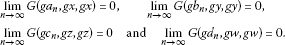(2.47)

Analogously, we show that(2.48)

Combining (2.47) and (2.48) yields that $\left(gx,gy,gz,gw\right)$ and $\left(gu,gv,gr,gl\right)$ are equal.

Since $gx=F\left(x,y,z,w\right)$, $gy=F\left(y,z,w,x\right)$, $gz=F\left(z,w,x,y\right)$, and $gz=F\left(z,w,x,y\right)$, by commutativity of F and g, we haveand

$g{w}^{\mathrm{\prime }}=g\left(gw\right)=g\left(F\left(w,x,y,z\right)\right)=F\left(gw,gx,gy,gz\right),$

where $gx={x}^{\mathrm{\prime }}$, $gy={y}^{\mathrm{\prime }}$, $gz={z}^{\mathrm{\prime }}$, and $gw={w}^{\mathrm{\prime }}$. Thus, $\left({x}^{\mathrm{\prime }},{y}^{\mathrm{\prime }},{z}^{\mathrm{\prime }},{w}^{\mathrm{\prime }}\right)$ is a quadruple coincidence point of F and g. Consequently, $\left(g{x}^{\mathrm{\prime }},g{y}^{\mathrm{\prime }},g{z}^{\mathrm{\prime }},g{z}^{\mathrm{\prime }}\right)$ and $\left(gx,gy,gz,gw\right)$ are equal. We deduce

$g{x}^{\mathrm{\prime }}=gx={x}^{\mathrm{\prime }},\phantom{\rule{2em}{0ex}}g{y}^{\mathrm{\prime }}=gy={y}^{\mathrm{\prime }}\phantom{\rule{1em}{0ex}}\text{and}\phantom{\rule{1em}{0ex}}g{z}^{\mathrm{\prime }}=gz={z}^{\mathrm{\prime }},\phantom{\rule{2em}{0ex}}g{w}^{\mathrm{\prime }}=gw={w}^{\mathrm{\prime }}.$

Therefore, $\left({x}^{\mathrm{\prime }},{y}^{\mathrm{\prime }},{z}^{\mathrm{\prime }},{w}^{\mathrm{\prime }}\right)$ is a quadruple common fixed point of F and g. Its uniqueness follows easily from (2.1). □

Example 2.1 Let $X=\mathbb{R}$ with a usual ordering. Define $G:X×X×X\to X$ by $G\left(x,y,z\right)=max\left\{|x-y|,|y-z|,|x-z|\right\}$. Let $g:X\to X$ and $F:X×X×X×X\to X$ be defined by

Take $\varphi :\left[0,+\mathrm{\infty }\right)\to \left[0,+\mathrm{\infty }\right)$ be given by $\varphi \left(t\right)=\frac{4}{3}t$ for all $t\in \left[0,+\mathrm{\infty }\right)$ and $\psi :\left[0,+\mathrm{\infty }\right)\to \left[0,+\mathrm{\infty }\right)$ by $\psi \left(t\right)=\frac{2}{3}t$ for all $t\in \left[0,+\mathrm{\infty }\right)$. Then

1. a.

$\left(X,G,\le \right)$ is a complete ordered G-metric space.

2. b.

For $x,y,z,w,u,v,s,t,a,b,c,d\in X$ with $gx\ge gu\ge ga$, $gy\le gv\le gb$, $gz\ge gh\ge gc$, and $gw\le gt\le gd$, we have the condition (2.1) of Theorem 2.1 satisfied.

3. c.

F and g have the mixed g-monotone property.

Proof To prove (b), given $x,y,z,w,u,v,s,t,a,b,c,d\in X$ with $gx\ge gu\ge ga$, $gy\le gv\le gb$, $gz\ge gs\ge gc$, and $gw\le gt\le gd$. Then

$|F\left(x,y,z,w\right)-F\left(u,v,s,t\right)|=\frac{1}{24}\left(\left(x-u\right)+\left(v-y\right)+\left(z-s\right)+\left(t-w\right)\right)$

but(2.49)

Similarly,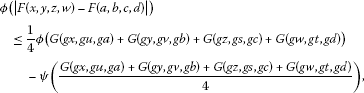(2.50)

and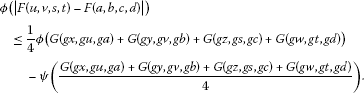(2.51)

Therefore,(2.52)

Hence,(2.53)

To prove (c), let $x,y,z,w\in X$. To show that $F\left(x,y,z,w\right)$ is g-monotone non-decreasing in x, let ${x}_{1},{x}_{2}\in X$ with $g{x}_{1}\le g{x}_{2}$. Then ${x}_{1}\le {x}_{2}$, and so ${x}_{1}-y+z-w\le {x}_{2}-y+z-w$. Hence, $F\left({x}_{1},y,z,w\right)\le F\left({x}_{2},y,z,w\right)$.

Therefore, $F\left(x,y,z,w\right)$ is g-monotone non-decreasing in x. Similarly, we may show that $F\left(x,y,z,w\right)$ is g-monotone non-decreasing in z.

To show that $F\left(x,y,z,w\right)$ is g-monotone non-increasing in y, let ${y}_{1},{y}_{2}\in X$ with $g{y}_{1}\le g{y}_{2}$, then ${y}_{1}\le {y}_{2}$. Hence, $x-{y}_{2}+z-w\le x-{y}_{1}+z-w$, so $F\left(x,{y}_{2},z,w\right)\le F\left(x,{y}_{1},z,w\right)$.

Therefore, $F\left(x,y,z,w\right)$ is g-monotone non-increasing in y. Similarly, we may show that $F\left(x,y,z,w\right)$ is g-monotone non-increasing in w.

Let ${x}_{o}={y}_{o}={z}_{o}={w}_{o}=0$. It is obvious that other hypotheses of Theorem 2.1 are satisfied. Thus, by Theorems 2.1 and 2.2, F and g have a unique quadruple common fixed point. Here, $\left(0,0,0,0\right)$ is the unique quadruple common fixed point of F and g. □

## 3 Application

In this part, we use previously obtained results to deduce some quadruple coincidence point results for mappings satisfying a contraction of integral type in a complete G-metric space. We first introduce some notations.

We denote by Γ the set of functions $\alpha :\left[0,+\mathrm{\infty }\right)\to \left[0,+\mathrm{\infty }\right)$ satisfying the following conditions:

1. (i)

α is a Lebesgue integrable mapping on each compact subset of $\left[0,+\mathrm{\infty }\right)$;

2. (ii)

for all $ϵ>0$, we have

${\int }_{0}^{ϵ}\alpha \left(s\right)\phantom{\rule{0.2em}{0ex}}ds>0;$
3. (iii)

α is sub-additive on each $\left[a,b\right]\subset \left[0,+\mathrm{\infty }\right)$, that is,

${\int }_{0}^{a+b}\alpha \left(t\right)\phantom{\rule{0.2em}{0ex}}dt\le {\int }_{0}^{a}\alpha \left(t\right)\phantom{\rule{0.2em}{0ex}}dt+{\int }_{0}^{b}\alpha \left(t\right)\phantom{\rule{0.2em}{0ex}}dt.$

Let $N\in \mathbf{N}$ be fixed. Let ${\left\{{\alpha }_{i}\right\}}_{1\le i\le N}$ be a family of N functions that belong to Γ. For all $t\ge 0$, we denote ${\left({I}_{i}\right)}_{i=1,\dots ,N}$ as follows:We have the following result.

Theorem 3.1 Let $\left(X,\le \right)$ be a partially ordered set and $\left(X,G\right)$ be a complete G-metric space. Suppose $F:{X}^{4}\to X$ and $g:X\to X$ are such that F is continuous and F has the mixed g-monotone property. Assume that there exist $\varphi \in \mathrm{\Phi }$ and $\psi \in \mathrm{\Psi }$ such that(3.1)

for any $x,y,z,w,u,v,s,t,a,b,c,d\in X$, for which $gx\ge gu\ge ga$, $gy\le gv\le gb$, $gz\ge gs\ge gc$, and $gw\le gt\le gd$. Also, suppose $F\left({X}^{4}\right)\subseteq g\left(X\right)$, g is continuous and commutes with F. If there exist ${x}_{0},{y}_{0},{z}_{0},{w}_{0}\in X$ such that $g{x}_{0}\le F\left({x}_{0},{y}_{0},{z}_{0},{w}_{0}\right)$, $g{y}_{0}\ge F\left({y}_{0},{z}_{0},{w}_{0},{x}_{0}\right)$, $g{z}_{0}\le F\left({z}_{0},{w}_{0},{x}_{0},{y}_{0}\right)$, and $g{w}_{0}\ge F\left({w}_{0},{x}_{0},{y}_{0},{z}_{0}\right)$, then there exist $x,y,z,w\in X$ such that

$F\left(x,y,z,w\right)=gx,\phantom{\rule{2em}{0ex}}F\left(y,z,w,x\right)=gy,\phantom{\rule{2em}{0ex}}F\left(z,w,x,y\right)=gz,\phantom{\rule{1em}{0ex}}\mathit{\text{and}}\phantom{\rule{1em}{0ex}}F\left(w,x,y,z\right)=gw,$

that is, F and g have a quadruple coincidence point.

Proof Take $\overline{\varphi }={I}_{N}\circ \varphi$ and $\overline{\psi }={I}_{N}\circ \psi$.

Note that the ${\left\{{\alpha }_{i}\right\}}_{1\le i=1,\dots ,N}$ are taken to be sub-additive on each $\left[a,b\right]\subset \left[0,+\mathrm{\infty }\right)$ in order to get

$\overline{\varphi }\left(a+b\right)\le \overline{\varphi }\left(a\right)+\overline{\varphi }\left(b\right).$

Moreover, it is easy to show that $\overline{\varphi }$ is continuous, non-decreasing and verifies $\overline{\varphi }\left(t\right)=0$ iff $t=0$.

We get that $\overline{\varphi }\in \mathrm{\Phi }$. Also, we can find that $\overline{\psi }\in \mathrm{\Psi }$. From (3.1), we have(3.2)

Now, applying Theorem 2.1, we obtain the desired result. □

## References

1. Dhage BC: Generalized metric space and mapping with fixed point. Bull. Calcutta Math. Soc. 1992, 84: 329–336.

2. Dhage BC: Generalized metric space and topological structure I. An. ştiinţ. Univ. “Al.I. Cuza” Iaşi, Mat. 2000, 46: 3–24.

3. Dhage BC: On generalized metric spaces and topological structure II. Pure Appl. Math. Sci. 1994, 40: 37–41.

4. Dhage BC: On continuity of mappings in D-metric spaces. Bull. Calcutta Math. Soc. 1994, 86: 503–508.

5. Mustafa Z, Sims B: Some remarks concerning D-metric spaces. Proceedings of the International Conferences on Fixed Point Theory and Applications 2003, 189–198. Valencia (Spain), July

6. Mustafa Z, Obiedat H, Awawdeh F: Some fixed point theorem for mappings on complete G -metric spaces. Fixed Point Theory Appl. 2008., 2008: Article ID 189870

7. Mustafa Z, Shatanawi W, Bataineh M: Existence of fixed point results in G -metric spaces. Int. J. Math. Math. Sci. 2009., 2009: Article ID 283028

8. Mustafa Z, Obiedat H: A fixed point theorem of Reich in G -metric spaces. CUBO 2010, 12(01):83–93. 10.4067/S0719-06462010000100008

9. Mustafa Z, Sims B: Fixed point theorems for contractive mappings in complete G -metric spaces. Fixed Point Theory Appl. 2009., 2009: Article ID 917175. doi:10.1155/2009/917175

10. Mustafa Z, Khandagjy M, Shatanawi W: Fixed point results on complete G -metric spaces. Studia Sci. Math. Hung. 2011, 48(3):304–319. doi:10.1556/SScMath.2011.1170

11. Mustafa Z, Awawdeh F, Shatanawi W: Fixed point theorem for expansive mappings in G -metric spaces. Int. J. Contemp. Math. Sci. 2010, 5: 49–52.

12. Obiedat H, Mustafa Z: Fixed point results on a nonsymmetric G -metric spaces. Jordan J. Math. Stat. 2010, 3(2):65–79.

13. Mustafa Z, Aydi H, Karapinar E: On common fixed points in G -metric spaces using (E.A) property. Comput. Math. Appl. 2012. doi:10.1016/j.camwa.2012.03.051

14. Mustafa Z: Common fixed points of weakly compatible mappings in G -metric spaces. Appl. Math. Sci. 2012, 6(92):4589–4600.

15. Mustafa Z: Some new common fixed point theorems under strict contractive conditions in G -metric spaces. J. Appl. Math. 2012., 2012: Article ID 248937. doi:10.1155/2012/248937

16. Rao KPR, Bhanu Lakshmi K, Mustafa Z: Fixed and related fixed point theorems for three maps in G -metric space. J. Adv. Dtud. Topol. 2012, 3(4):12–19.

17. Shatanawi W: Fixed point theory for contractive mappings satisfying Φ-maps in G -metric spaces. Fixed Point Theory Appl. 2010., 2010: Article ID 181650

18. Shatanawi W: Some fixed point theorems in ordered G -metric spaces and applications. Abstr. Appl. Anal. 2011., 2011: Article ID 126205

19. Aydi H, Damjanović B, Samet B, Shatanawi W: Coupled fixed point theorems for nonlinear contractions in partially ordered G -metric spaces. Math. Comput. Model. 2011. doi:10.1016/j.mcm.2011.05.059

20. Shatanawi W, Samet B: On $\left(\psi ,\varphi \right)$-weakly contractive condition in partially ordered metric spaces. Comput. Math. Appl. 2011, 62: 3204–3214. 10.1016/j.camwa.2011.08.033

21. Bhaskar TG, Lakshmikantham V: Fixed point theorems in partially ordered metric spaces and applications. Nonlinear Anal. 2006, 65: 1379–1393. 10.1016/j.na.2005.10.017

22. Lakshmikantham V, Ciric L: Couple fixed point theorems for nonlinear contractions in partially ordered metric spaces. Nonlinear Anal. 2009, 70: 4341–4349. 10.1016/j.na.2008.09.020

23. Choudhury BS, Maity P: Coupled fixed point results in generalized metric spaces. Math. Comput. Model. 2011, 54: 73–79. 10.1016/j.mcm.2011.01.036

24. Karapinar E: Couple fixed point theorems for nonlinear contractions in cone metric spaces. Comput. Math. Appl. 2010, 59: 3656–3668. 10.1016/j.camwa.2010.03.062

25. Lakshmikantham V, Ćirić Lj: Coupled fixed point theorems for nonlinear contractions in partially ordered metric spaces. Nonlinear Anal. 2009, 70: 4341–4349. 10.1016/j.na.2008.09.020

26. Luong NV, Thuan NX: Coupled fixed points in partially ordered metric spaces and applications. Nonlinear Anal. 2011, 72: 983–992.

27. Nashine, HK, Shatanawi, W: Coupled common fixed point theorems for a pair of commuting mappings in partially ordered complete metric spaces. Comput. Math. Appl. (to appear)

28. Sabetghadam F, Masiha HP, Sanatpour AH: Some coupled fixed point theorems in cone metric spaces. Fixed Point Theory Appl. 2009., 2009: Article ID 125426

29. Samet B: Coupled fixed point theorems for a generalized Meir-Keeler contraction in partially ordered metric spaces. Nonlinear Anal. 2010, 72: 4508–4517. 10.1016/j.na.2010.02.026

30. Samet B, Vetro C: Coupled fixed point, f -invariant set and fixed point of N -order. Ann. Funct. Anal. 2010, 1(2):46–56.

31. Samet B, Yazidi H: Coupled fixed point theorems in partially ordered ε -chainable metric spaces. TJMCS 2010, 1(3):142–151.

32. Sedghi S, Altun I, Shobe N: Coupled fixed point theorems for contractions in fuzzy metric spaces. Nonlinear Anal. 2010, 72: 1298–1304. 10.1016/j.na.2009.08.018

33. Shatanawi W: Some common coupled fixed point results in cone metric spaces. Int. J. Math. Anal. 2010, 4: 2381–2388.

34. Shatanawi W: Partially ordered cone metric spaces and coupled fixed point results. Comput. Math. Appl. 2010, 60: 2508–2515. 10.1016/j.camwa.2010.08.074

35. Shatanawi W, Samet B, Abbas M: Coupled fixed point theorems for mixed monotone mappings in ordered partial metric spaces. Math. Comput. Model. 2012, 55: 680–687. 10.1016/j.mcm.2011.08.042

36. Shatanawi W: Coupled fixed point theorems in generalized metric spaces. Hacet. J. Math. Stat. 2011, 40(3):441–447.

37. Shatanawi W, Abbas M, Nazir T: Common coupled coincidence and coupled fixed point results in two generalized metric spaces. Fixed Point Theory Appl. 2011, 2011: 80. 10.1186/1687-1812-2011-80

38. Shatanawi W: Partially ordered cone metric spaces and coupled fixed point results. Comput. Math. Appl. 2010, 60: 2508–2515. 10.1016/j.camwa.2010.08.074

39. Berinde V, Borcut M: Tripled fixed point theorems for contractive type mappings in partially ordered metric spaces. Nonlinear Anal. 2011, 74(15):4889–4897. 10.1016/j.na.2011.03.032

40. Aydi H, Karapınar E, Postolache M: Tripled coincidence point theorems for weak φ -contractions in partially ordered metric spaces. Fixed Point Theory Appl. 2012, 2012: 44. 10.1186/1687-1812-2012-44

41. Karapınar E, Luong NV: Quadruple fixed point theorems for nonlinear contractions. Comput. Math. Appl. 2012. doi:10.1016/j.camwa.2012.02.061

42. Karapınar, E: Quartet fixed point for nonlinear contraction. http://arxiv.org/abs/1106.5472

43. Karapınar E: Quadruple fixed point theorems for weak ϕ -contractions. ISRN Math. Anal. 2011., 2011: Article ID 989423

44. Karapınar E, Berinde V: Quadruple fixed point theorems for nonlinear contractions in partially ordered metric spaces. Banach J. Math. Anal. 2012, 6(1):74–89.

45. Karapınar E: A new quartet fixed point theorem for nonlinear contractions. JP J. Fixed Point Theory Appl. 2011, 6(2):119–135.

46. Karapinar E, Shatanawi W, Mustafa Z: Quadruple fixed point theorems under nonlinear contractive conditions in partially ordered metric spaces. J. Appl. Math. 2012., 2012: Article ID 951912. doi:10.1155/2012/951912

47. Mustafa Z, Aydi H, Karapinar E: Mixed g -monotone property and quadruple fixed point theorems in partial ordered metric space. Fixed Point Theory Appl. 2012., 2012: Article ID 71. doi:10.1186/1687–1812–2012–71

48. Mustafa Z, Sims B: A new approach to generalized metric spaces. J. Nonlinear Convex Anal. 2006, 7(2):289–297.

## Author information

Authors

### Competing interests

The author declares that they have no competing interests.

## Rights and permissions

Reprints and Permissions

Mustafa, Z. Mixed g-monotone property and quadruple fixed point theorems in partially ordered G-metric spaces using $\left(\varphi -\psi \right)$ contractions. Fixed Point Theory Appl 2012, 199 (2012). https://doi.org/10.1186/1687-1812-2012-199

• Accepted:

• Published:

• DOI: https://doi.org/10.1186/1687-1812-2012-199

### Keywords